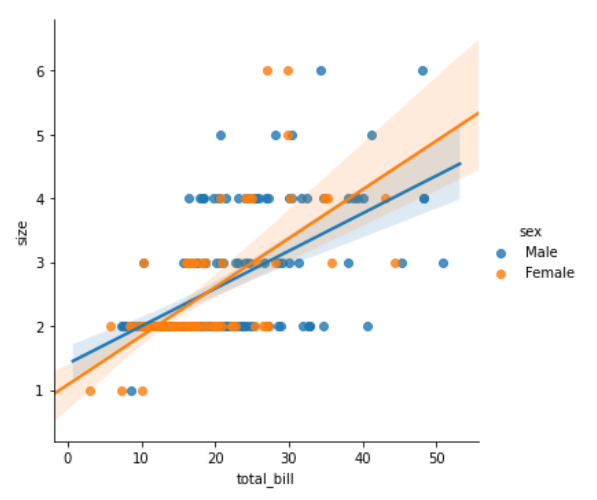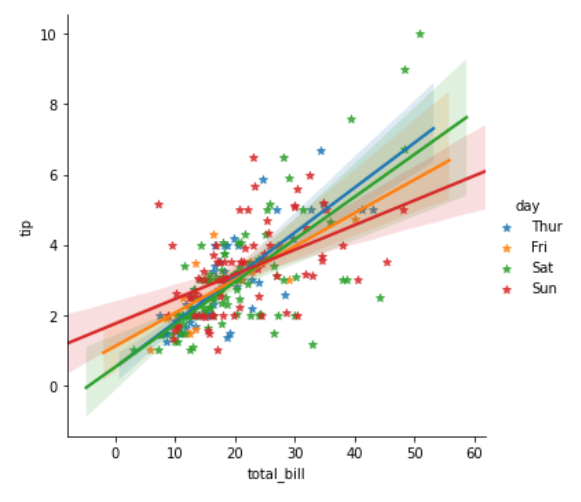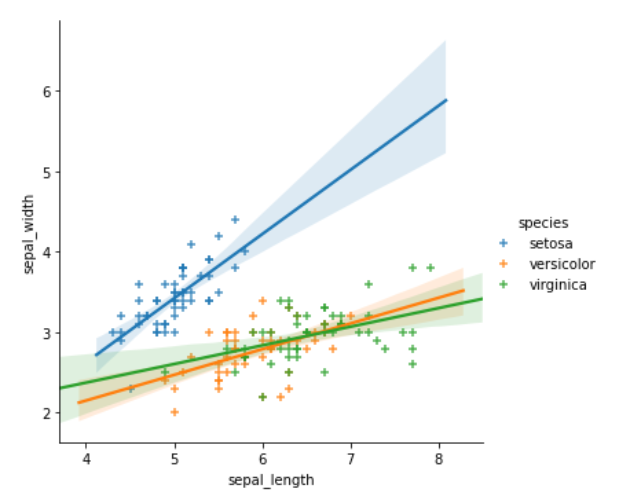GeeksforGeeks App
Open AppBrowser
Continue

# How To Add Regression Line Per Group with Seaborn in Python?

In this article, we will learn how to add a regression line per group with Seaborn in Python. Seaborn has multiple functions to form scatter plots between two quantitative variables. For example, we can use lmplot() function to make the required plot.

## What is Regression Line?

A regression line is just one line that most closely fits the info (in terms of getting the littlest overall distance from the road to the points). Statisticians call this system for locating the best-fitting line an easy rectilinear regression analysis using the smallest amount squares method.

### Steps Required

1. Import Library.
2. Import or create data.
3. Use lmplot method. This method is used to add a regression line per group by simply adding the hue parameter with the categorical variable name.
4. Use different arguments for better visualization.

Example 1:

## Python3

 `# import libraries``import` `seaborn`` ` `# load data``tip ``=` `seaborn.load_dataset(``'tips'``)`` ` `# use lmplot``seaborn.lmplot(x``=``"total_bill"``,``               ``y``=``"size"``,``               ``hue``=``"sex"``,``               ``data``=``tip)`

Output:Example 2:

## Python3

 `# import libraries``import` `seaborn`` ` `# load data``tip ``=` `seaborn.load_dataset(``'tips'``)`` ` `# use lmplot``seaborn.lmplot(x``=``"total_bill"``,``               ``y``=``"tip"``,``               ``hue``=``"day"``,``               ``markers``=``'*'``,``               ``data``=``tip)`

Output:Example 3:

## Python3

 `# import libraries``import` `seaborn`` ` `# load data``iris ``=` `seaborn.load_dataset(``'iris'``)`` ` `# use lmplot``seaborn.lmplot(x``=``"sepal_length"``,``               ``y``=``"sepal_width"``,``               ``hue``=``"species"``,``               ``markers``=``'+'``,``               ``data``=``iris)`

Output:My Personal Notes arrow_drop_up